Courses

# Binary Search Trees MCQ - 1

## 20 Questions MCQ Test RRB JE for Computer Science Engineering | Binary Search Trees MCQ - 1

Description
This mock test of Binary Search Trees MCQ - 1 for Computer Science Engineering (CSE) helps you for every Computer Science Engineering (CSE) entrance exam. This contains 20 Multiple Choice Questions for Computer Science Engineering (CSE) Binary Search Trees MCQ - 1 (mcq) to study with solutions a complete question bank. The solved questions answers in this Binary Search Trees MCQ - 1 quiz give you a good mix of easy questions and tough questions. Computer Science Engineering (CSE) students definitely take this Binary Search Trees MCQ - 1 exercise for a better result in the exam. You can find other Binary Search Trees MCQ - 1 extra questions, long questions & short questions for Computer Science Engineering (CSE) on EduRev as well by searching above.
QUESTION: 1

### What is the worst case time complexity for search, insert and delete operations in a general Binary Search Tree?

Solution:

In skewed Binary Search Tree (BST), all three operations can take O(n). See the following example BST and operations.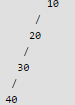Search 40.
Delete 40
Insert 50.

QUESTION: 2

### In delete operation of BST, we need inorder successor (or predecessor) of a node when the node to be deleted has both left and right child as non-empty. Which of the following is true about inorder successor needed in delete operation?

Solution:

Let X be the node to be deleted in a tree with root as 'root'. There are three cases for deletion 1) X is a leaf node: We change left or right pointer of parent to NULL (depending upon whether X is left or right child of its parent) and we delete X 2) One child of X is empty: We copy values of non-empty child to X and delete the non-empty child 3) Both children of X are non-empty: In this case, we find inorder successor of X. Let the inorder successor be Y. We copy the contents of Y to X, and delete Y. Sp we need inorder successor only when both left and right child of X are not empty. In this case, the inorder successor Y can never be an ancestor of X. In this case, the inorder successor is the leftmost node in right subtree of X. Since it is leftmost node, the left child of Y must be empty.

QUESTION: 3

### We are given a set of n distinct elements and an unlabeled binary tree with n nodes. In how many ways can we populate the tree with the given set so that it becomes a binary search tree? (GATE CS 2011)

Solution:

There is only one way. The minimum value has to go to the leftmost node and the maximum value to the rightmost node. Recursively, we can define for other nodes.

QUESTION: 4

How many distinct binary search trees can be created out of 4 distinct keys?

Solution:
QUESTION: 5

Which of the following traversal outputs the data in sorted order in a BST?

Solution:

Inorder traversal of a BST outputs data in sorted order. Read here for details.

QUESTION: 6

Suppose the numbers 7, 5, 1, 8, 3, 6, 0, 9, 4, 2 are inserted in that order into an initially empty binary search tree. The binary search tree uses the usual ordering on natural numbers. What is the in-order traversal sequence of the resultant tree?

Solution:

In-order traversal of a BST gives elements in increasing order. So answer c is correct without any doubt.

QUESTION: 7

The following numbers are inserted into an empty binary search tree in the given order: 10, 1, 3, 5, 15, 12, 16. What is the height of the binary search tree (the height is the maximum distance of a leaf node from the root)? (GATE CS 2004)

Solution:

Constructed binary search tree will be..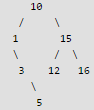QUESTION: 8

The preorder traversal sequence of a binary search tree is 30, 20, 10, 15, 25, 23, 39, 35, 42. Which one of the following is the postorder traversal sequence of the same tree?

Solution:

The following is the constructed tree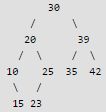QUESTION: 9

Consider the following Binary Search Tree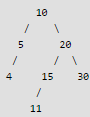Q.
If we randomly search one of the keys present in above BST, what would be the expected number of comparisons?

Solution:

Expected number of comparisons = (1*1 + 2*2 + 3*3 + 4*1)/7 = 18/7 = 2.57

QUESTION: 10

Which of the following traversals is sufficient to construct BST from given traversals 1) Inorder 2) Preorder 3) Postorder

Solution:

When we know either preorder or postorder traversal, we can construct the BST. Note that we can always sort the given traversal and get the inorder traversal. Inorder traversal of BST is always sorted.

QUESTION: 11

Consider the following code snippet in C. The function print() receives root of a Binary Search Tree (BST) and a positive integer k as arguments.

// A BST node
struct node {
int data;
struct node *left, *right;
};
int count = 0;

void print(struct node *root, int k)
{
if (root != NULL && count <= k)
{
print(root->right, k);
count++;
if (count == k)
printf("%d ", root->data);
print(root->left, k);
}
}

Q. What is the output of print(root, 3) where root represent root of the following BST.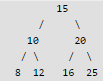Solution:

The code mainly finds out k'th largest element in BST, see K’th Largest Element in BSTfor details.

QUESTION: 12

Consider the same code as given in above question. What does the function print() do in general? The function print() receives root of a Binary Search Tree (BST) and a positive integer k as arguments.

// A BST node
struct node {
int data;
struct node *left, *right;
};
int count = 0;

void print(struct node *root, int k)
{
if (root != NULL && count <= k)
{
print(root->right, k);
count++;
if (count == k)
printf("%d ", root->data);
print(root->left, k);
}
}

Solution:

The function basically does reverse inorder traversal of the given Binary Search Tree. The reverse inorder traversal produces data in reverse sorted order. Whenever a nod is visited, count is incremented by 1 and data of a node is printed only when count becomes k.

QUESTION: 13

You are given the postorder traversal, P, of a binary search tree on the n elements 1, 2, ..., n. You have to determine the unique binary search tree that has P as its postorder traversal. What is the time complexity of the most efficient algorithm for doing this?

Solution:

One important thing to note is, it is Binary Search Tree, not Binary Tree. In BST, inorder traversal can always be obtained by sorting all keys.

QUESTION: 14

Suppose we have a balanced binary search tree T holding n numbers. We are given two numbers L and H and wish to sum up all the numbers in T that lie between L and H. Suppose there are m such numbers in T. If the tightest upper bound on the time to compute the sum is O(nalogb n + mclogd n), the value of a + 10b + 100c + 1000d is ____.

Solution:

int getSum(node *root, int L, int H)
{
// Base Case
if (root == NULL)
return 0;
if (root->key < L)
return getSum(root->right, L, H);
if (root->key > H)
return getSum(root->left, L, H)
if (root->key >= L && root->key <=H)
return getSum(root->left, L, H) + root->key + getSum(root->right, L, H);
}

The above always takes O(m + Logn) time. Note that the code first traverses across height to find the node which lies in range.  Once such a node is found, it recurs for left and right children. Two recursive calls are made only if the node is in range. So for every node that is in range, we make at most one extra call (here extra call means calling for a node that is not in range).

QUESTION: 15

Let T(n) be the number of different binary search trees on n distinct elements. Then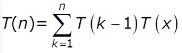where x is

Solution:

The idea is to make a key root, put (k-1) keys in one subtree and remaining n-k keys in other subtree. A Binary Search Tree (BST) is a tree in which all the nodes follow the below-mentioned properties −

• The left sub-tree of a node has a key less than or equal to its parent node's key.
1. The right sub-tree of a node has a key greater than to its parent node's key.

Now construction binary search trees from n distinct number- Lets for simplicity consider n distinct numbers as first n natural numbers (starting from 1) If n=1 We have only one possibility, therefore only 1 BST. If n=2 We have 2 possibilities , when smaller number is root and bigger number is the right child or second when the bigger number is root and smaller number as left child.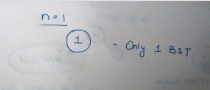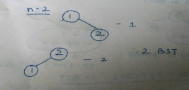If n=3 We have 5 possibilities. Keeping each number first as root and then arranging the remaining 2 numbers as in case of n=2.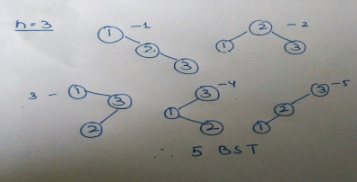n=4 We have 14 possibilities. Taking each number as root and arranging smaal numbers as left subtree and larger numbers as right subtree.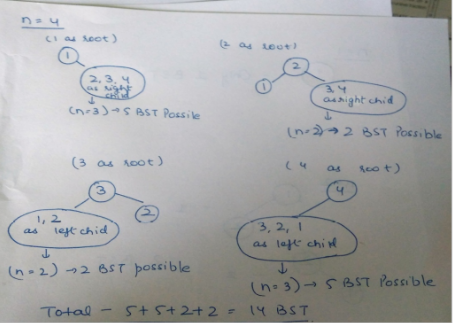Thus we can conclude that with n distinct numbers, if we take ‘k’ as root then all the numbers smaller than k will left subtree and numbers larger than k will be right subtree where the the right subtree and left subtree will again be constructed recursively like the root. Therefore,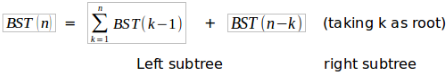QUESTION: 16

What are the worst-case complexities of insertion and deletion of a key in a binary search tree?

Solution:

The time taken by search, insert and delete on a BST is always proportional to height of BST. Height may become O(n) in worst case.

QUESTION: 17

While inserting the elements 71, 65, 84, 69, 67, 83 in an empty binary search tree (BST) in the sequence shown, the element in the lowest level is

Solution:

Here is The Insertion Algorithm For a Binary Search Tree :

Insert(Root,key)
{
if(Root is NULL)
Create a Node with value as key and return
Else if(Root.key <= key)                                     Insert(Root.left,key)
Else
Insert(Root.right,key)
}

Creating the BST one by one using the above algorithm in the below given image :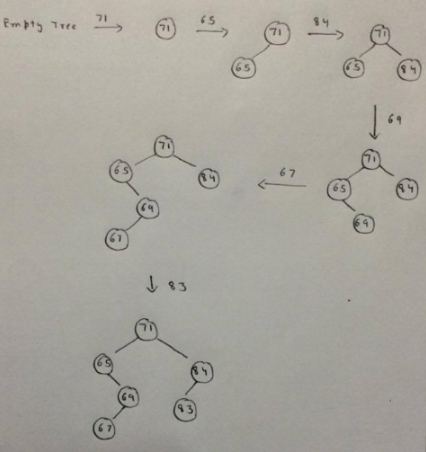QUESTION: 18

The number of ways in which the numbers 1, 2, 3, 4, 5, 6, 7 can be inserted in an empty binary search tree, such that the resulting tree has height 6, is _____________ Note: The height of a tree with a single node is 0. [This question was originally a Fill-in-the-Blanks question]

Solution:

To get height 6, we need to put either 1 or 7 at root. So count can be written as T(n) = 2*T(n-1) with T(1) = 1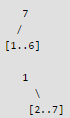Therefore count is 26 = 64 Another Explanation: Consider these cases, 1 2 3 4 5 6 7 1 2 3 4 5 7 6 1 7 6 5 4 3 2 1 7 6 5 4 2 3 7 6 5 4 3 2 1 7 6 5 4 3 1 2 7 1 2 3 4 5 6 7 1 2 3 4 6 5 For height 6, we have 2 choices. Either we select the root as 1 or 7. Suppose we select 7. Now, we have 6 nodes and remaining height = 5. So, now we have 2 ways to select root for this sub-tree also. Now, we keep on repeating the same procedure till remaining height = 1 For this last case also, we have 2 ways. Therefore, total number of ways = 26= 64

QUESTION: 19

Suppose that we have numbers between 1 and 100 in a binary search tree and want to search for the number 55. Which of the following sequences CANNOT be the sequence of nodes examined?

Solution:

In BST on right side of parent number should be greater than it, but in C after 47, 43 appears that is wrong.

QUESTION: 20

When searching for the key value 60 in a binary search tree, nodes containing the key values 10, 20, 40, 50, 70 80, 90 are traversed, not necessarily in the order given. How many different orders are possible in which these key values can occur on the search path from the root to the node containing the value 60?

Solution:

There are two set of values, smaller than 60 and greater than 60. Smaller values 10, 20, 40 and 50 are visited, means they are visited in order. Similarly, 90, 80 and 70 are visited in order.
= 7!/(4!3!)
= 35